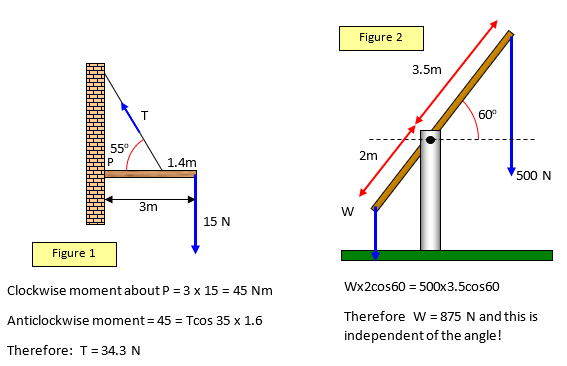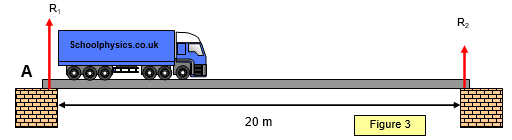# Moments 2

The following two diagrams show further applications of the principle of moments. The second diagram is pespecially interesting as it shows that W in this case is independent of the angle of the beam.Example problems
A truck is driven across a uniform bridge as shown in Figure 3. The truck has a mass of 4000 kg and the bridge is 20 m long and has a mass of 5000 kg.(a) what is the total reaction at the supports
(b) what is the reaction (R1 and R2 ) at each support when the centre of mass of the truck is:
(i) 5 m from end A
(ii) 12 m from end A

(a) total reaction at the supports = [5000 + 4000]g = 9000g
(b) (i) moments about end A: R2 x 20 = 4000g x 5 + 5000g x 10 = 70 000g  R2 = 3500g
Therefore R1 = 9000g – 3500g = 5500g
(ii) moments about end A: R2 x 20 = 4000g x 12 + 5000g x 10 = 98 000g     R2 = 4900g
Therefore R1 = 9000g – 4900g = 4100g

A VERSION IN WORD IS AVAILABLE ON THE SCHOOLPHYSICS USB